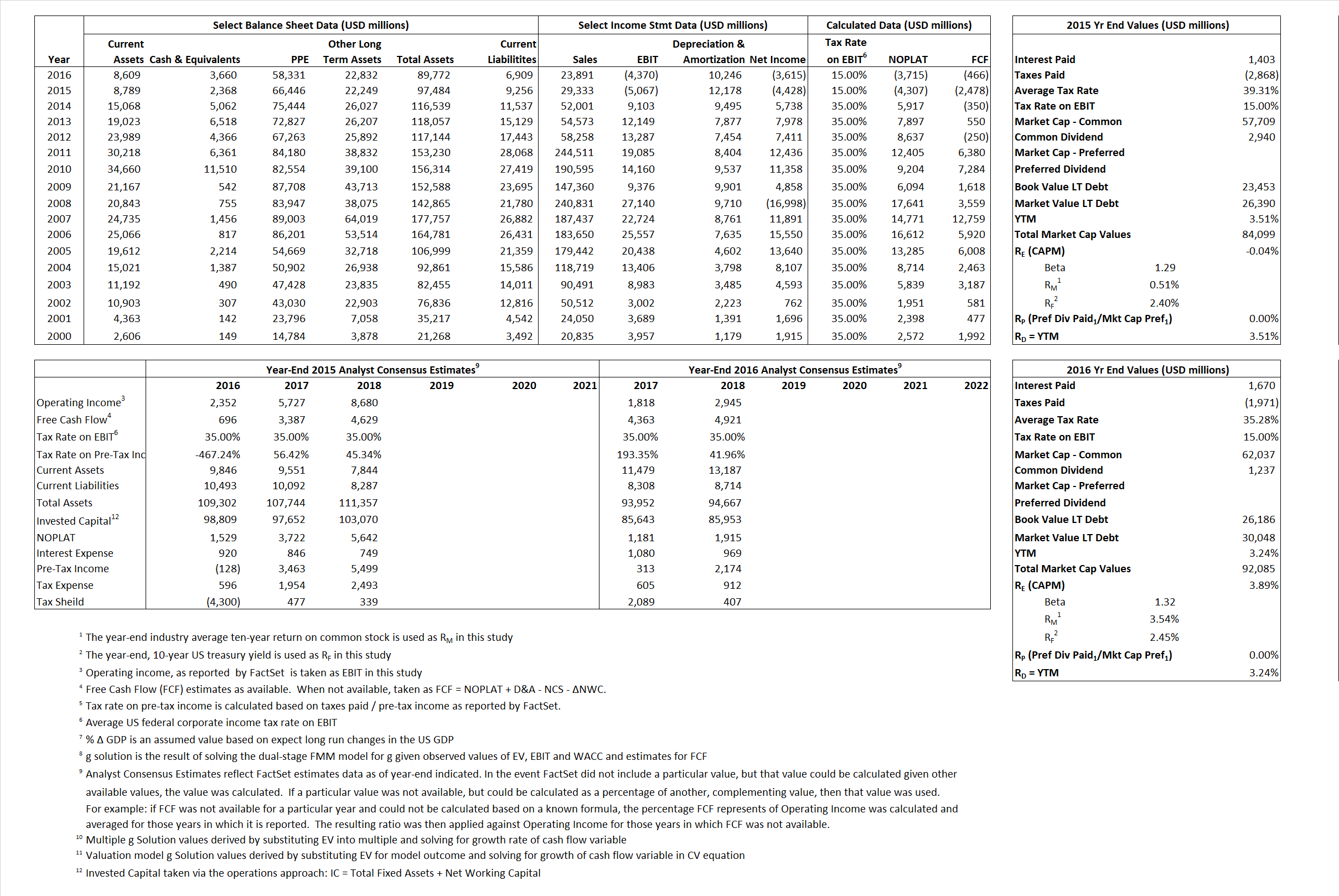# ConocoPhillips

## Analyst Listing

The following analysts provide coverage for the subject firm as of May 2016:

 Broker Analyst Analyst Email Scotia Howard Weil Blake Fernandez blake.fernandez@scotiabank.com Cowen & Company Charles Robertson charles.robertson@cowen.com Evercore ISI Douglas Terreson doug.terreson@evercoreisi.com Credit Suisse Edward Westlake edward.westlake@credit-suisse.com Piper Jaffray Guy Baber guy.a.baber@simmonspjc.com Alembic Global Advisors James Sullivan james.sullivan@alembicglobal.com Jefferies Jason Gammel jason.gammel@jefferies.com Nomura Research Lloyd Byrne lloyd.byrne@nomura.com Wolfe Research Paul Sankey psankey@wolferesearch.com Raymond James Pavel Molchanov pavel.molchanov@raymondjames.com Wells Fargo Securities Roger D. Read roger.read@wellsfargo.com Deutsche Bank Research Ryan Todd ryan.todd@db.com RBC Capital Markets Scott Hanold scott.hanold@rbccm.com

## Primary Input Data## Derived Input Data

### Equational Form

Net Operating Profit Less Adjusted Taxes NOPLAT (4,307)  (3,715)$NOPLAT\, =\, EBIT\, x\, (1 \,-\, Avg \,\,Tax\,\, Rate\,\, on\,\, EBIT)$
Free Cash Flow FCF (2,478) (466)$FCF\,=NOPLAT\,+\,Non-Cash\,Expenses-\Delta NWC\,-\,NCS$
Tax Shield TS 552 589$TS\,=\,Interest\,\,Paid\,\,x\,\, Avg \,\,Tax\,\,Rate\,\, on\,\, Pre-Tax\,\, Income$
Invested Capital IC 88,228 82,863$IC\,=\,Fixed\,\,Operating\,\,Assets\,\,+\,\,Net\,\, Working\,\, Capital$
Return on Invested Capital ROIC -4.88% -4.48%$ROIC\,=\,\frac { NOPLAT }{ IC }$
Net Investment NetInv (4,596) 4,881$NetInv\,=\,{ {IC}_{1}}-{{IC}_{0}}+Depreciation$
Investment Rate IR  106.71% -131.40%$IR\,=\,\frac {NetInv}{NOPLAT}$
Weighted Average Cost of Capital
WACCMarket 0.64%  3.30%$WACC\,=\,\frac { E }{ V } { R }_{ E }\,+\,\frac { P }{ V } { R }_{ P }\,+\,\frac { D }{ V } { R }_{ D }\left( 1- Avg\,\, Tax\,\,Rate\,\,on\,\,Pre-Tax\,\,Income \right)$
WACCBook  8.85%    8.64%
Enterprise value
EVMarket 81,731 88,425$EV\,=\,Market\,\,Cap\,\,Equity\,+\,\,Long\,\,Term\,\,Debt\,-\,Cash$
EVBook   78,794    84,563
Long-Run Growth
g = IR x ROIC
-5.21%  5.89% Long-run growth rates of the income variable are used in the Continuing Value portion of the valuation models.
g = %$\Delta$ GDP   2.50%    2.50%
Margin from Operations M  -17.27%  -18.29%$M\,\,=\,\,\frac{EBIT}{SALES}$
Depreciation/Amortization Rate D  171.26%  174.37%$D\,\,=\,\,\frac{D+A}{EBITDA}$

## Valuation Multiple Outcomes

The outcomes presented in this study are the result of original input data, derived data, and synthesized inputs.

### model g solution

12/31/2015 12/31/2016 12/31/2015 12/31/2016 12/31/2015 12/31/2016

EV/SALES$\frac {EV}{Sales} \,= \,\frac{ROIC\, -\, g}{ROIC\,(WACC\,-\,g)}\,(1\,-\,T)\,(M)$

2.79  3.70  -74.37%  119.32%  -35.05%  59.40%

EV/EBITDA$\frac {EV}{EBITDA} \,= \,\frac{ROIC\, -\, g}{ROIC\,(WACC\,-\,g)}\,(1\,-\,T)\,(1\,-\,D)$

11.49 15.05 -74.37% 119.32% -35.05% 59.40%

EV/NOPLAT$\frac {EV}{NOPLAT} \,= \,\frac{ROIC\, -\, g}{ROIC\,(WACC\,-\,g)}$

-18.98 -23.81 -74.37% 119.32% -35.05% 59.40%

EV/FCFOPS$\frac {EV}{FCF_{OPS}} \,= \,\frac{ROIC\, -\, g}{ROIC\,(WACC\,-\,g)}\,(1\,-\,T)$

-32.98 -189.75 -74.37% 119.32% -35.05% 59.40%

EV/EBIT$\frac {EV}{EBIT} \,= \,\frac{ROIC\, -\, g}{ROIC\,(WACC\,-\,g)}\,(1\,-\,T)$

-16.13 -20.23 -74.37% 119.32% -35.05% 59.40%

EV/IC$\frac {EV}{IC} \,= \,\frac{ROIC\, -\, g}{WACC\,-\,g}$

0.93 1.07 -74.37% 119.32% -35.05% 59.40%